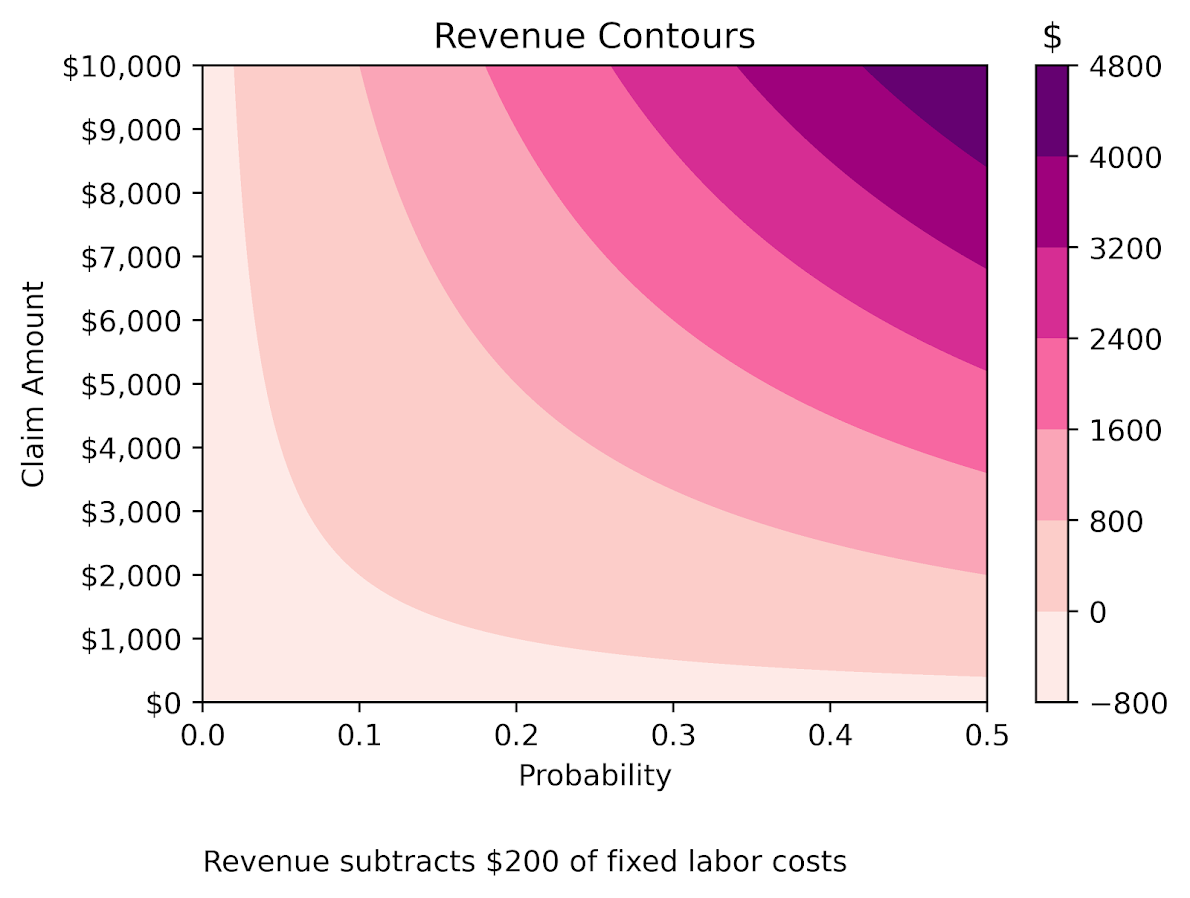# Filled contour plot in python

I’ve been making a chart that looks similar to this for a few different projects at work, so figured a quick blog post to show the notes of it would be useful.

So people often talk about setting a decision threshold to turn a predicted probability into a binary yes/no decision. E.g. do I do some process to this observation if the probability is 20%, 30%, 60%, etc. If you can identify the costs and benefits of making particular decisions, you can set a simple threshold to make that decision. For example, say you are sending adverts in the mail for a product. If the person buys the product, your company makes \$50, and the advert only costs \$1 to send. In this framework, if you have a predictive model for the probability the advert will be successful, then your decision threshold will look like this:

``\$50*probability - \$1``

So in this case you need the predicted probability to be above 2% to have an expected positive return on the investment of sending the advert. So if you have a probability of 10% for 2000 customers, you would expect to make `2000 * (50*0.1 - 1) = 8000`. The probabilities you get from your predictive model can be thought of as in the long run averages. Any single advert may be a bust, but if your model is right and you send out a bunch, you should make this much money in the end. (If you have a vector of varying probabilities, in R code the estimated revenue will then look like `prob <- runif(2000,0,0.1); pover <- prob > 0.02; sum( (50*prob - 1)*pover )`.)

But many of the decisions I work with are not a single number in the benefits column. I am working with medical insurance claims data at HMS, and often determining models to audit those claims in some way. In this framework, it is more important to audit a high dollar claim than a lower dollar claim, even if the higher dollar value claim has a lower probability. So I have been making the subsequent filled contour plot I am going to show in the next section to illustrate this.

# python contour plot

The code snippet is small enough to just copy-paste entirely. First, I generate data over a regular grid to illustrate different claim amounts and then probabilities. Then I use `np.meshgrid` to get the data in the right shape for the contour plot. The revenue estimates are then simply the probability times the claims amount, minus some fixed (often labor to audit the claim) cost. After that is is just idiosyncratic matplotlib code to make a nice filled contour.

``````# Example of making a revenue contour plot
import matplotlib.pyplot as plt
from matplotlib.ticker import StrMethodFormatter
import numpy as np

n = 500 #how small grid cells are
prob = np.linspace(0,0.5,n)
dollar = np.linspace(0,10000,n)
#np.logspace(0,np.log10(10000),n) #if you want to do logged

# Generate grid
X, Y = np.meshgrid(prob, dollar)

# Example generating revenue
fixed = 200
Rev = (Y*X) - fixed

fig, ax = plt.subplots()
CS = ax.contourf(X, Y, Rev, cmap='RdPu')
clb = fig.colorbar(CS)
#clb.ax.set_xlabel('Revenue') #Abit too wide
clb.ax.set_title('dollar') #html does not like the dollar sign
ax.set_xlabel('Probability')
ax.set_ylabel('Claim Amount')
ax.yaxis.set_major_formatter(StrMethodFormatter('\${x:,.0f}'))
plt.title('Revenue Contours')
plt.xticks(np.arange(0,0.6,0.1))
plt.yticks(np.arange(0,11000,1000))
plt.annotate('Revenue subtracts \$200 of fixed labor costs',
(0,0), (0, -50),
xycoords='axes fraction',
textcoords='offset points', va='top')
#plt.savefig('RevContour.png',dpi=500,bbox_inches='tight')
plt.show()``````The color bar does nice here out of the box. Next up in my personal learning will be how to manipulate color bars a bit more. Here I may want to either use a mask to not show negative expected returns, or a diverging color scheme (e.g. blue for negative returns).